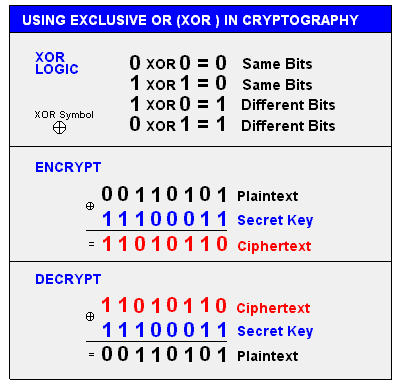exclusive or

(redirected from Bit xor)
Also found in: Dictionary.

exclusive or

[ik¦sklü·siv ′ȯr]
(computer science)
An instruction which performs the “exclusive or” operation on a bit-by-bit basis for its two operand words, usually storing the result in one of the operand locations. Abbreviated XOR.
(mathematics)
A logic operator which has the property that if P is a statement and Q is a statement, then P exclusive or Q is true if either but not both statements are true, false if both are true or both are false.
McGraw-Hill Dictionary of Scientific & Technical Terms, 6E, Copyright © 2003 by The McGraw-Hill Companies, Inc.

exclusive or

(logic)
(XOR, EOR) /X or, E or/ A two-input Boolean logic function whose result is true if one input is true and the other is false. The truth table is

A | B | A xor B --+---+-------- F | F | F F | T | T T | F | T T | T | F

The output is thus true if the inputs are not equal. If one input is false, the other is passed unchanged whereas if one input is true, the other is inverted.

In Boolean algebra, exclusive or is often written as a plus in a circle: "⊕". The circle may be omitted suggesting addition modulo two.

In digital logic, an exclusive or logic gate is drawn like a normal inclusive or gate but with a curved line across both inputs: exclusive or gate.
XOR in CryptographyThis example uses XOR and the same "secret key" to encrypt and decrypt. Although XOR logic may be used, secret key algorithms are much more sophisticated than this. See encryption algorithm.